# MAFS.6.NS.1.1Archived StandardExport Print
Interpret and compute quotients of fractions, and solve word problems involving division of fractions by fractions, e.g., by using visual fraction models and equations to represent the problem. For example, create a story context for (2/3) ÷ (3/4) and use a visual fraction model to show the quotient; use the relationship between multiplication and division to explain that (2/3) ÷ (3/4) = 8/9 because 3/4 of 8/9 is 2/3. (In general, (a/b) ÷ (c/d) = ad/bc.) How much chocolate will each person get if 3 people share 1/2 lb of chocolate equally? How many 3/4-cup servings are in 2/3 of a cup of yogurt? How wide is a rectangular strip of land with length 3/4 mi and area 1/2 square mi?

### Remarks

Examples of Opportunities for In-Depth Focus

This is a culminating standard for extending multiplication and division to fractions.

Fluency Expectations or Examples of Culminating Standards

Students interpret and compute quotients of fractions and solve word problems involving division of fractions by fractions. This completes the extension of operations to fractions.
General Information
Subject Area: Mathematics
Domain-Subdomain: The Number System
Cluster: Level 2: Basic Application of Skills & Concepts
Cluster: Apply and extend previous understandings of multiplication and division to divide fractions by fractions. (Major Cluster) -

Clusters should not be sorted from Major to Supporting and then taught in that order. To do so would strip the coherence of the mathematical ideas and miss the opportunity to enhance the major work of the grade with the supporting clusters.

Date of Last Rating: 02/14
Status: State Board Approved - Archived
Assessed: Yes
Test Item Specifications

• Assessment Limits :
At least the divisor or dividend needs to be a non-unit fraction. Dividing a unit fraction by a whole number or vice versa (e.g.,÷ ?? or ?? ÷, where a is a whole number) is below grade level.
• Calculator :

No

• Context :

Allowable

Sample Test Items (4)
• Test Item #: Sample Item 1
• Question:

An expression is shown.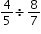What is the value of the expression?

• Difficulty: N/A
• Type: EE: Equation Editor

• Test Item #: Sample Item 2
• Question:

An expression is shown.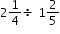What is the value of the expression?

• Difficulty: N/A
• Type: EE: Equation Editor

• Test Item #: Sample Item 3
• Question:

A rectangular plot of land has an area of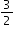square kilometers and a length of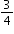kilometer.

What is the width of the plot of land?

• Difficulty: N/A
• Type: EE: Equation Editor

• Test Item #: Sample Item 4
• Question:

Which question can be answered using the expression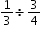?

• Difficulty: N/A
• Type: MC: Multiple Choice

## Related Courses

This benchmark is part of these courses.
1205010: M/J Grade 6 Mathematics (Specifically in versions: 2014 - 2015, 2015 - 2022, 2022 and beyond (current))
1205020: M/J Accelerated Mathematics Grade 6 (Specifically in versions: 2014 - 2015, 2015 - 2020, 2020 - 2022, 2022 and beyond (current))
1204000: M/J Foundational Skills in Mathematics 6-8 (Specifically in versions: 2014 - 2015, 2015 - 2022, 2022 and beyond (current))
7812015: Access M/J Grade 6 Mathematics (Specifically in versions: 2014 - 2015, 2015 - 2018, 2018 - 2022, 2022 and beyond (current))
7912110: Fundamental Explorations in Mathematics 1 (Specifically in versions: 2013 - 2015, 2015 - 2017 (course terminated))

## Related Access Points

Alternate version of this benchmark for students with significant cognitive disabilities.

## Related Resources

Vetted resources educators can use to teach the concepts and skills in this benchmark.

## Educational Game

Fraction Quiz:

Test your fraction skills by answering questions on this site. This quiz asks you to simplify fractions, convert fractions to decimals and percentages, and answer algebra questions involving fractions. You can even choose difficulty level, question types, and time limit.

Type: Educational Game

## Formative Assessments

Models of Fraction Division:

Students are asked to explain the relationship between a fraction division word problem and either a visual model or an equation.

Type: Formative Assessment

Fraction Division:

Students are asked to complete two fraction division problems – one with fractions and one with mixed numbers.

Type: Formative Assessment

Contextualizing Fraction Division:

Students are asked to write a story context for a given fraction division problem.

Type: Formative Assessment

Juicing Fractions:

Students are asked to write and evaluate a numerical expression involving division of fractions and mixed numbers to model and solve a word problem.

Type: Formative Assessment

## Lesson Plans

Gr. 6 Lesson 2-A Drop in the Bucket:

Students will recognize the concern for water quantity and water flow to the Everglades through a demonstration and activity.

Type: Lesson Plan

Dividing Fractions: Increasing Procedural Skill:

This is a lesson designed to help students develop procedural skill in dividing fractions. During this lesson students will discuss prior knowledge of the concept of dividing fractions and will solve problems independently and in pairs using the standard algorithm. The lesson also incorporates music and poster-making to reinforce the learning objective. This lesson was originally designed for a 6th grade Intensive Math class, and should only be used following lessons where students have developed a solid conceptual understanding of dividing fractions. This lesson may take up to 3 days depending on how much practice and extra activities your students require.

Type: Lesson Plan

The Price is Right:

In this activity the students will apply their knowledge of mathematical calculations to solve a real-world problem. They will analyze a collection of shipping boxes to determine which box will ship the most for the \$100 allowed.

Type: Lesson Plan

Fancy Fractions Catering Company Project:

Fancy Fractions Catering Company will be hosting a party and need your help to make it happen! Your help is needed to find out how much of each ingredient is needed to feed 200 people and the most economical way of doing this (Brand name brands or store brand). You also have the option of omitting up to three ingredients from the recipe.

Type: Lesson Plan

Dividing Fractions:

In this lesson students will explore the different methods available for dividing fractions through a student-based investigation. The teacher will facilitate the discussion, but the students will discover the different methods on their own or with a partner as they work through the different steps.

Type: Lesson Plan

Dividing Fractions (Part 1) - Tackling Word Problems:

This lesson allows the students to explore the foundation for dividing fractions as well as correctly solving word problems involving division of fractions. It includes the use of the Philosophical Chairs activity and numerical solutions. Group activities are included to foster cooperative learning.

Type: Lesson Plan

Dividing by Fractions Discovery:

This lesson allows students to derive an algorithm for dividing fractions using visual fraction models and equations to represent the problem.

Type: Lesson Plan

Wheeling Around:

Students are taught the vocabulary used for circles and apply a formula to find the circumference of a bicycle wheel.

Type: Lesson Plan

Running to School, Variation 2:

Students are asked to solve a distance problem involving fractions.

Making Hot Cocoa, Variation 1:

Students are asked to solve a fraction division problem using both a visual model and the standard algorithm within a real-world context.

Making Hot Cocoa, Variation 2:

Students are asked a series of questions involving a fraction and a whole number within the context of a recipe. Students are asked to solve a problem using both a visual model and the standard algorithm.

Running to School, Variation 3:

Students are asked to solve a distance problem involving fractions. The purpose of this task is to help students extend their understanding of division of whole numbers to division of fractions, and given the simple numbers used, it is most appropriate for students just learning about fraction division because it lends itself easily to a pictorial solution.

Traffic Jam:

Students are asked to use fractions to determine how many hours it will take a car to travel a given distance.

Video Game Credits:

Students are asked to use fractions to determine how long a video game can be played.

The purpose of this task is to help students get a better understanding of multiplying and dividing using fractions.

Cup of Rice:

The purpose of this task is to help give students a better understanding of multiplying and dividing fractions.

Dan’s Division Strategy:

The purpose of this task is to help students explore the meaning of fraction division and to connect it to what they know about whole-number division. Students are asked to explain why the quotient of two fractions with common denominators is equal to the quotient of the numerators of those fractions.

Drinking Juice, Variation 2:

This task builds on a fifth grade fraction multiplication task, "Drinking Juice." This task uses the identical context, but asks the corresponding "Number of Groups Unknown" division problem. See "Drinking Juice, Variation 3" for the "Group Size Unknown" version.

Drinking Juice, Variation 3:

Students are asked to solve a fraction division problem using a visual model and the standard algorithm.

How Many _______ Are In. . . ?:

This instructional task requires that the students model each problem with some type of fractions manipulatives or drawings. This could be pattern blocks, student or teacher-made fraction strips, or commercially produced fraction pieces. At a minimum, students should draw pictures of each. The above problems are meant to be a progression which require more sophisticated understandings of the meaning of fractions as students progress through them.

How Many Containers in One Cup / Cups in One Container?:

The purpose of this problem is to help students deepen their understanding of the meaning of fractions and fraction division and to see that they get the same answer using standard algorithm as they do just reasoning through the problem. These two fraction division tasks use the same context and ask "How much in one group?" but require students to divide the fractions in the opposite order. Students struggle to understand which order one should divide in a fraction division context, and these two tasks give them an opportunity to think carefully about the meaning of fraction division.

## Professional Development

Fractions, Percents, and Ratios, Part A: Models for Multiplication and Division of Fractions:

This professional development module shows teachers how to use area models to understand multiplication and division of fractions.

Type: Professional Development

## Student Center Activity

Students can practice answering mathematics questions on a variety of topics. With an account, students can save their work and send it to their teacher when complete.

Type: Student Center Activity

## Tutorial

Dividing Mixed Numbers:

In this tutorial, you will see how mixed numbers can be divided.

Type: Tutorial

## Video/Audio/Animation

Reciprocals and Divisions of Fractions:

When working with fractions, divisions can be converted to multiplication by the divisor's reciprocal. This chapter explains why.

Type: Video/Audio/Animation

## STEM Lessons - Model Eliciting Activity

Fancy Fractions Catering Company Project:

Fancy Fractions Catering Company will be hosting a party and need your help to make it happen! Your help is needed to find out how much of each ingredient is needed to feed 200 people and the most economical way of doing this (Brand name brands or store brand). You also have the option of omitting up to three ingredients from the recipe.

## MFAS Formative Assessments

Contextualizing Fraction Division:

Students are asked to write a story context for a given fraction division problem.

Fraction Division:

Students are asked to complete two fraction division problems – one with fractions and one with mixed numbers.

Juicing Fractions:

Students are asked to write and evaluate a numerical expression involving division of fractions and mixed numbers to model and solve a word problem.

Models of Fraction Division:

Students are asked to explain the relationship between a fraction division word problem and either a visual model or an equation.

## Student Resources

Vetted resources students can use to learn the concepts and skills in this benchmark.

## Educational Game

Fraction Quiz:

Test your fraction skills by answering questions on this site. This quiz asks you to simplify fractions, convert fractions to decimals and percentages, and answer algebra questions involving fractions. You can even choose difficulty level, question types, and time limit.

Type: Educational Game

Running to School, Variation 2:

Students are asked to solve a distance problem involving fractions.

Making Hot Cocoa, Variation 1:

Students are asked to solve a fraction division problem using both a visual model and the standard algorithm within a real-world context.

Making Hot Cocoa, Variation 2:

Students are asked a series of questions involving a fraction and a whole number within the context of a recipe. Students are asked to solve a problem using both a visual model and the standard algorithm.

Running to School, Variation 3:

Students are asked to solve a distance problem involving fractions. The purpose of this task is to help students extend their understanding of division of whole numbers to division of fractions, and given the simple numbers used, it is most appropriate for students just learning about fraction division because it lends itself easily to a pictorial solution.

Traffic Jam:

Students are asked to use fractions to determine how many hours it will take a car to travel a given distance.

Video Game Credits:

Students are asked to use fractions to determine how long a video game can be played.

Dan’s Division Strategy:

The purpose of this task is to help students explore the meaning of fraction division and to connect it to what they know about whole-number division. Students are asked to explain why the quotient of two fractions with common denominators is equal to the quotient of the numerators of those fractions.

Drinking Juice, Variation 2:

This task builds on a fifth grade fraction multiplication task, "Drinking Juice." This task uses the identical context, but asks the corresponding "Number of Groups Unknown" division problem. See "Drinking Juice, Variation 3" for the "Group Size Unknown" version.

Drinking Juice, Variation 3:

Students are asked to solve a fraction division problem using a visual model and the standard algorithm.

How Many _______ Are In. . . ?:

This instructional task requires that the students model each problem with some type of fractions manipulatives or drawings. This could be pattern blocks, student or teacher-made fraction strips, or commercially produced fraction pieces. At a minimum, students should draw pictures of each. The above problems are meant to be a progression which require more sophisticated understandings of the meaning of fractions as students progress through them.

## Student Center Activity

Students can practice answering mathematics questions on a variety of topics. With an account, students can save their work and send it to their teacher when complete.

Type: Student Center Activity

## Tutorial

Dividing Mixed Numbers:

In this tutorial, you will see how mixed numbers can be divided.

Type: Tutorial

## Video/Audio/Animation

Reciprocals and Divisions of Fractions:

When working with fractions, divisions can be converted to multiplication by the divisor's reciprocal. This chapter explains why.

Type: Video/Audio/Animation

## Parent Resources

Vetted resources caregivers can use to help students learn the concepts and skills in this benchmark.

Running to School, Variation 2:

Students are asked to solve a distance problem involving fractions.

Making Hot Cocoa, Variation 1:

Students are asked to solve a fraction division problem using both a visual model and the standard algorithm within a real-world context.

Making Hot Cocoa, Variation 2:

Students are asked a series of questions involving a fraction and a whole number within the context of a recipe. Students are asked to solve a problem using both a visual model and the standard algorithm.

Running to School, Variation 3:

Students are asked to solve a distance problem involving fractions. The purpose of this task is to help students extend their understanding of division of whole numbers to division of fractions, and given the simple numbers used, it is most appropriate for students just learning about fraction division because it lends itself easily to a pictorial solution.

Traffic Jam:

Students are asked to use fractions to determine how many hours it will take a car to travel a given distance.

Video Game Credits:

Students are asked to use fractions to determine how long a video game can be played.

The purpose of this task is to help students get a better understanding of multiplying and dividing using fractions.

Dan’s Division Strategy:

The purpose of this task is to help students explore the meaning of fraction division and to connect it to what they know about whole-number division. Students are asked to explain why the quotient of two fractions with common denominators is equal to the quotient of the numerators of those fractions.

Drinking Juice, Variation 2:

This task builds on a fifth grade fraction multiplication task, "Drinking Juice." This task uses the identical context, but asks the corresponding "Number of Groups Unknown" division problem. See "Drinking Juice, Variation 3" for the "Group Size Unknown" version.